## Friday, April 1, 2016

### Further updates due to modification of `direction="leftwards"` option in `plotSimmap`

A couple of days ago I posted an update to the way that left-facing trees are plotting using phytools. Before, they were plotted as right-facing trees, but with the axis flipped to run right to left. This created problems with some functions designed to interact with the plotted object, such as `nodelabels` in ape.

In the updated version, left-facing trees are plotted from zero a the tips to the maximum tree depth - this the absyssa runs from left to right as normal. This is in line with what the core S3 plotting method `plot.phylo` of ape also does.

One of the consequences of this update is that some other functions that use `plotSimmap` internally also have to be updated. Among these are included `contMap` and `densityMap`. The changes are relatively minor. In particular, the legend increased from right to left instead of the reverse, as in this example: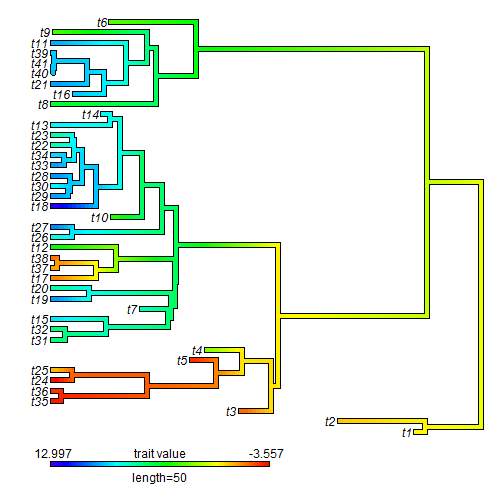I have now fixed this small thing, although other functions are still affected.

``````library(phytools)
tree
``````
``````##
## Phylogenetic tree with 26 tips and 25 internal nodes.
##
## Tip labels:
##  A, B, C, D, E, F, ...
##
## Rooted; includes branch lengths.
``````
``````x
``````
``````##           A           B           C           D           E           F
##  1.90762587  1.57924943  2.15505369  1.88567603  1.21106074  0.08040664
##           G           H           I           J           K           L
##  0.06688040 -2.87016896 -2.16359495 -1.75453560 -1.70905693 -2.40875267
##           M           N           O           P           Q           R
## -1.56404914 -0.73540639  0.10856695 -0.34097916  0.27630258 -0.91287290
##           S           T           U           V           W           X
## -2.25318016 -2.25348990 -1.74692303 -0.92818524 -1.17855084 -0.14342536
##           Y           Z
## -0.80488661 -1.28504659
``````
``````obj<-contMap(tree,x,plot=FALSE)
plot(obj,legend=1)
``````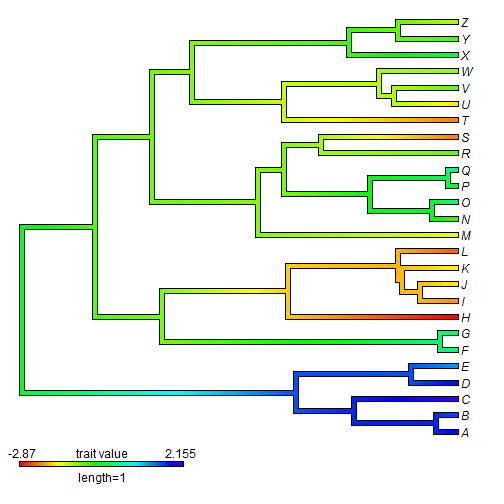``````plot(obj,legend=1,direction="leftwards")
``````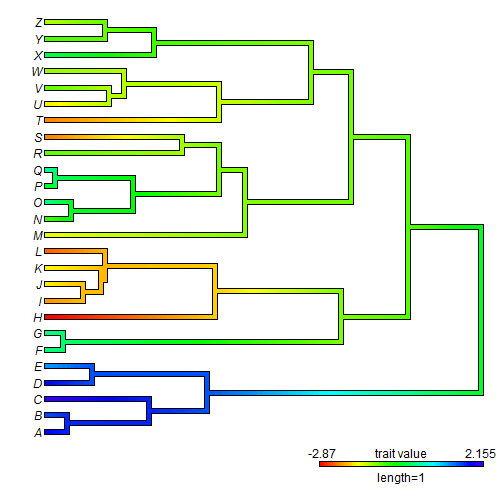As before we can take advantage of this new feature to have time run backwards from the right to left (i.e., “time before present”). We do this using `direction="leftwards"`, but `xlim>xlim`.

``````plot(obj,legend=1,direction="leftwards",mar=c(4.1,0.1,0.1,0.1),sig=2,
xlim=c(3,0)) ## flip x dimensions
axis(1)
``````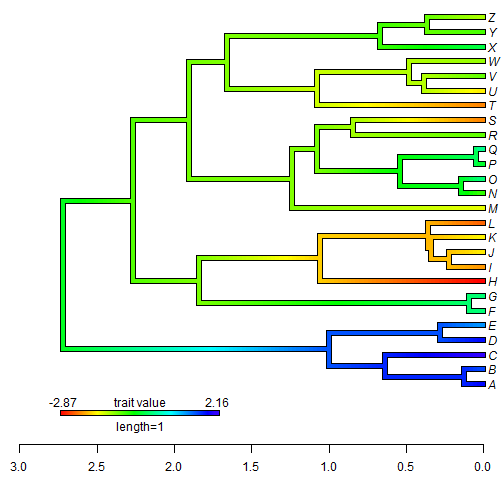This also applies to stochastic mapping and `densityMap`:

``````y
``````
``````## A B C D E F G H I J K L M N O P Q R S T U V W X Y Z
## b b b a a a a b a a a b b b b a a b a a a a b b a a
## Levels: a b
``````
``````trees<-make.simmap(tree,y,nsim=100)
``````
``````## make.simmap is sampling character histories conditioned on the transition matrix
##
## Q =
##           a         b
## a -1.004826  1.004826
## b  1.004826 -1.004826
## (estimated using likelihood);
## and (mean) root node prior probabilities
## pi =
##   a   b
## 0.5 0.5
``````
``````## Done.
``````
``````obj<-densityMap(trees,plot=FALSE)
``````
``````## sorry - this might take a while; please be patient
``````
``````plot(obj,outline=TRUE,lwd=5)
``````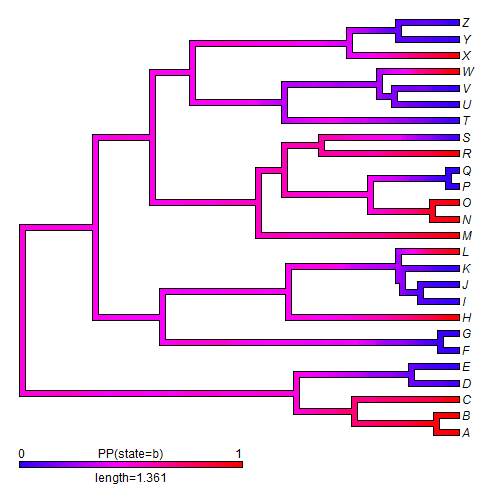``````plot(obj,outline=TRUE,lwd=5,direction="leftwards")
``````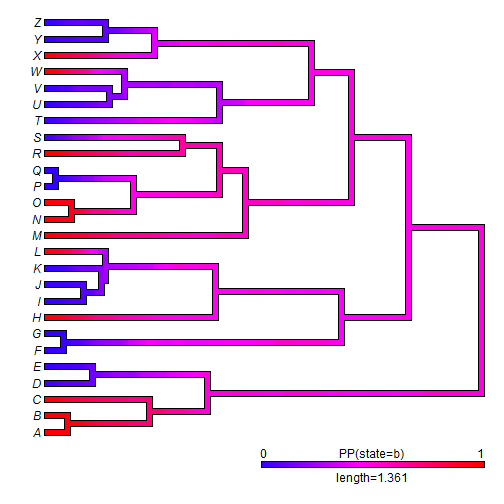Unfortunately, some functions are still not fully functional for leftward plotting, such as `dotTree`:

``````dotTree(tree,y,colors=setNames(c("blue","red"),c("a","b")),ftype="i")
``````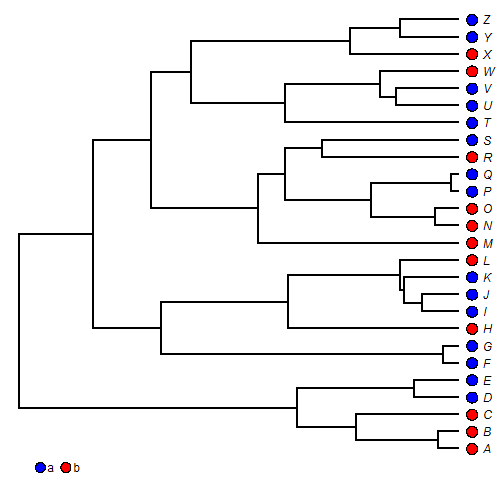``````dotTree(tree,y,colors=setNames(c("blue","red"),c("a","b")),ftype="i",
direction="leftwards")
``````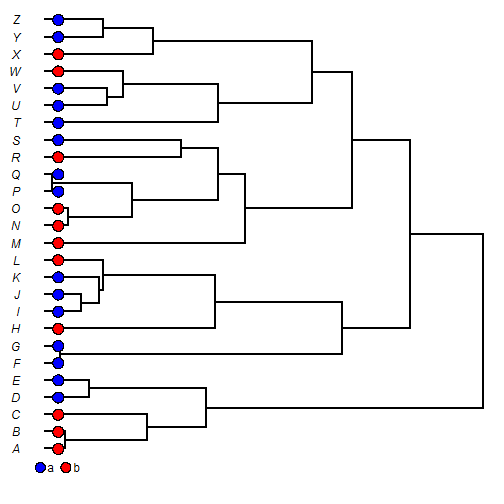This update, should you choose to obtain it, can be installed from GitHub using devtools:

``````library(devtools)
install_github("liamrevell/phytools")
``````

The data for this example were simulated as follows:

``````tree<-pbtree(n=26,tip.label=LETTERS)
x<-fastBM(tree)
Q<-matrix(c(-1,1,1,-1),2,2)
rownames(Q)<-colnames(Q)<-letters[1:2]
y<-sim.history(tree,Q)\$states
y<-as.factor(y)
``````